Next: 4.2 Parameter Modeling Up: 4. Device Simulation and Previous: 4. Device Simulation and

Subsections

# 4.1 Semiconductor Equations

## 4.1.1 Poisson's and Continuity Equation

In macroscopic semiconductor device modeling, Poisson's equation and the continuity equations play a fundamental role. Poisson's equation, one of the basic equations in electrostatics, is derived from the Maxwell's equation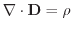and the material relation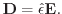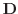stands for the electric displacement field,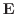for the electric field,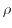is the charge density, and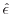is the permittivity tensor. Using the electrostatic potential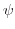with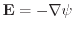leads to Poisson's equation(4.1)

Even for high frequencies, where the wavelengths are typically much smaller than the device dimension, the quasi stationary approximation used to justify Poisson's equation is still valid. Also the permittivity tensoris considered time invariant in this derivation. In isotropic materials like silicon the permittivity can be additionally approximated by the scalar value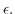Furthermore the permittivity is often considered to be constant within a material segment.

In semiconductors the charge density is commonly split into fixed charges which are in particular ionized acceptors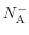and donors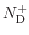and into free charges which are electronsand holes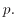Including the acceptors, donors, electrons, and holes into (4.1), Poisson's equation can be written as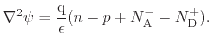(4.2)

The right hand side of (4.2) becomes zero in ideal oxides, and Poisson's equation reduces to the Laplace equation. However, the existence of oxide charges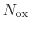has to be considered, which yields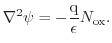(4.3)

Additionally, at interfaces and surfaces, the interface trap concentration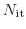has to be considered.

The continuity equation, can be also derived from Maxwell's equations and reads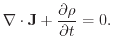(4.4)

The current density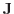is split into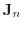and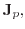for the contribution of electrons and holes, respectively. The electron and hole concentrations are written asand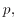respectively, and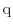is used to represent the elementary charge. By introducing the recombination rate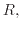two separate continuity equations can be formulated as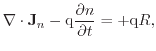(4.5)

for electrons and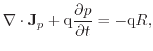(4.6)

for holes. The separation into two equations allows independent transport modeling of the carrier types. The rate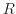represents the net rate, which is zero in thermal equilibrium, following that generation and recombination are balanced. Generation and recombination rates of electrons and holes are expressed using physically or empirically based recombination models , which will be presented in Section 4.2. The equations (4.5) and (4.6) can alternatively be derived from Boltzmann's transport equation using the method of moments .

## 4.1.2 Carrier Transport Equations

A semiclassical description of carrier transport is given by Boltzmann's transport equation (BTE) which describes the evolution of the distribution function in the six-dimensional phase space (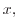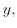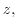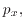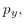). Unfortunately, analytical solutions exist only for very simple configurations. One popular approach for solving the BTE in arbitrary structures is the Monte Carlo method  which gives highly accurate results. However, due to the statistical nature of the Monte Carlo method solutions are computationally very expensive. Due to the good agreement with experiments  results are often used as reference for the evaluation of simpler models. Alternatively, the spherical harmonics expansion (SHE) method as a deterministic numerical solution method of the BTE was presented already in the 1960s . However, it has just been recently, that an efficiently use on real devices has been realized .

Device simulations on an engineering level require simpler transport equations which can be solved for complex structures within reasonable time. One method to perform this simplification is to consider only moments of the distribution function . Depending on the number of moments considered in the model, different transport equations can be derived. The use of the first two moments, leads to the well known drift-diffusion model, a widely used approach for modeling carrier transport.

## 4.1.3 The Drift-Diffusion Model

Beside the derivation of the drift-diffusion by the method of moments , it is also possible using basic principles of irreversible thermodynamics . The resulting electron and hole current relations contain at least two components caused by carrier drift and carrier diffusion. Additionally, the gradient of the lattice temperature (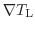) acts as a driving force on the free carriers leading to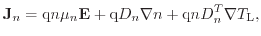(4.7)

and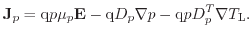(4.8)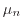and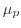represent the carrier electron and hole mobility,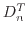andthe thermal diffusion coefficients, and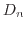and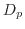the diffusivity, respectively. The diffusivities are commonly expressed via the mobility invoking the Einstein relation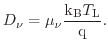(4.9)

The indexstands forandrespectively, while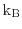is the Boltzmann constant. The Einstein relation is strictly valid only in equilibrium .

The equations (4.7) and (4.8) together with (4.5), (4.6), and (4.2) form the drift-diffusion model which was first presented by Van Roosbroeck in the year 1950 . Rigorous derivations from the BTE show that many simplifications are required to obtain the drift-diffusion equations as will be shown. Simplifications include, for example, the assumption of a single parabolic band structure and the cold Maxwellian carrier distribution function. This assumes the carrier temperature equal to the lattice temperature. Nevertheless, due to its simplicity and its excellent numerical properties, the drift-diffusion equations have become the workhorse for most TCAD applications.

## 4.1.4 Higher-Order Transport Models

To obtain a better approximation of the BTE, higher-order transport models can be derived using more than just the first two moments . The most prominent models beside the drift-diffusion model are the energy-transport/hydrodynamic models which use three or four moments. These models are based on the work of Stratton  and Bløtekjær . A detailed review is given in . In addition to the quantities used in the drift-diffusion model, the energy flux and the carrier temperatures are introduced as independent variables. Also new balance and flux equations are required, which introduce additional transport parameters. The carrier energy distribution function is here modeled using the heated Maxwellian distribution. Modeling of carrier mobility and impact-ionization benefit from more accurate models based on the carrier temperatures rather than the electric field. This advantage is caused by the non-local behavior of the average energy with respect to the electric field and becomes especially relevant for small device structures. This context is explained in Fig. 4.2.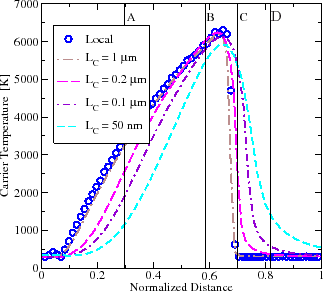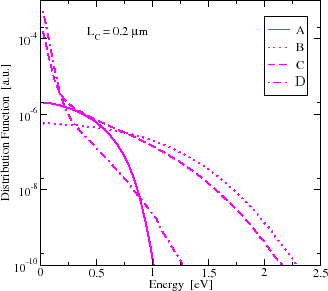More detailed examinations in the far sub-micron area show that describing the energy distribution function using only the carrier concentration and the carrier temperature is still not sufficient for specific problems which depend on high energy tails (see Fig. 4.2). Hot carrier modeling in small structures, for example, which is based on accurate modeling of the high-energy part of the distribution function would require more complex models. Also the reliability modeling benefits of the detailed knowledge of the distribution function (more on this is highlighted in Chapter 6). One method for improving the approximation of the distribution function is the six moments method . It introduces the kurtosis, which is the deviation of the distribution function from the heated Maxwellian.

It is evident that higher-order transport models give a closer solution of the BTE and therefore lead to a better agreement between simulation results and measurements of real devices. This effect is especially relevant for small structures, where non-local effects gain importance (see Fig. 4.2). Simulation results with drift-diffusion in deep sub-micrometer structures therefore seem to be very questionable .

The high-voltage devices considered in this work are relatively large. Hence, a rather high number of mesh points is required for a proper discretization. In combination with more elaborative transport equations, this leads to a higher computational time. Additionally, the convergence properties degrade significantly for higher moments models . However, the relatively large dimensions of the high-voltage devices justify the use of the drift-diffusion model in this work.

In case advanced transport models have to be solved in complex devices, it is also possible to combine different transport equations in one simulation. To accomplish this, the semiconductor domain must be partitioned into separate segments. One segment must contain the critical areas, e.g. the channel area. Hence, the higher-order transport equations are solved for this segment, and the drift-diffusion model is used for the remaining ones. Here, it is essential to define proper boundary conditions between the segments .Next: 4.2 Parameter Modeling Up: 4. Device Simulation and Previous: 4. Device Simulation and

O. Triebl: Reliability Issues in High-Voltage Semiconductor Devices